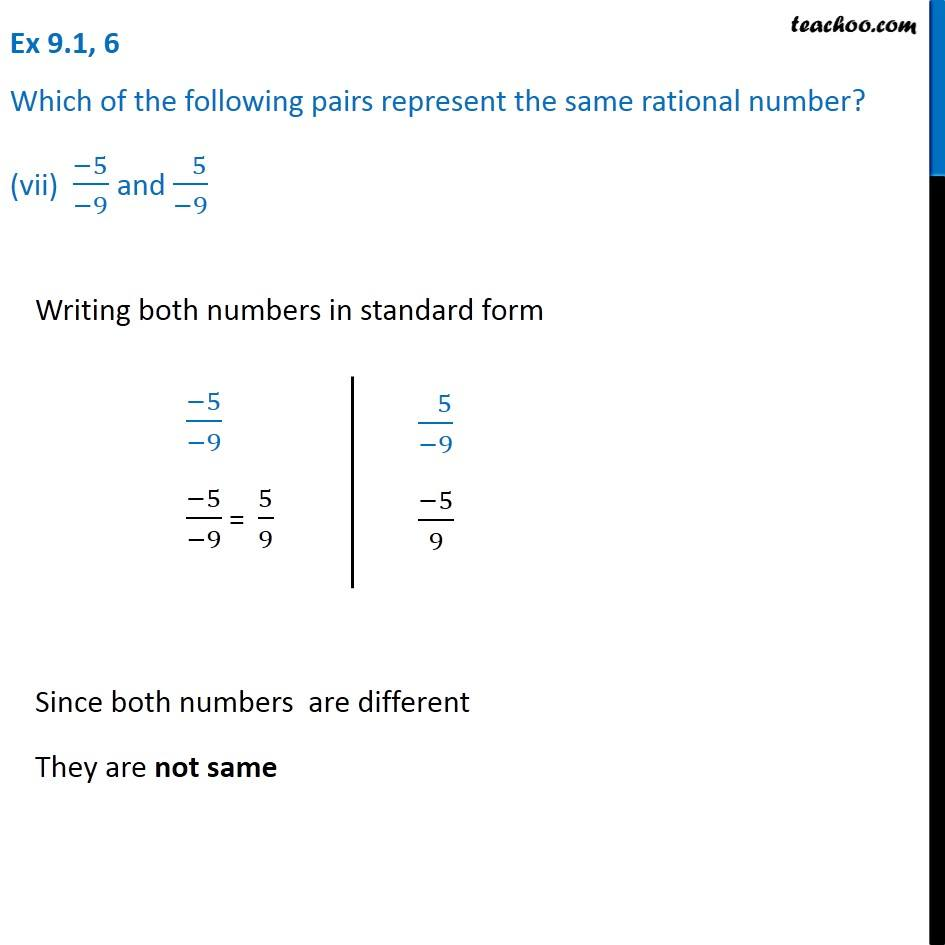Ex 9.1

Chapter 9 Class 7 Rational Numbers
Serial order wiseGet live Maths 1-on-1 Classs - Class 6 to 12

### Transcript

Ex 9.1, 6 Which of the following pairs represent the same rational number? (vii) (−5)/(−9) and ( 5)/(−9) Writing both numbers in standard form (−5)/(−9) (−5)/(−9) = 5/9 ( 5)/(−9) (−5)/9 Since both numbers are different They are not same